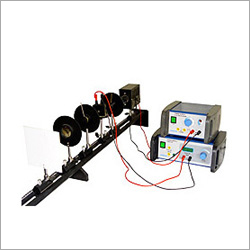0Physics Lab Kit
Product Description
Physics Lab Kit
We have been offering a wide range of higher science education physics lab kit which is customized as per the specifications of our clients. These physics lab kit is a combination of sophisticated technology and streamlined production process and include
• Kerr Effect Experiment Kit
• Newton's Ring Experiment Kit
• Fresnel Bi prism Experiment Kit
• Spectroscopy Experiment Kit
• Michelson Interferometer
• Electronic Plug-In Kit
• Milikan Oil Drop Experiment
• Measurement of Basic constants length, weight ,time
• Modulus of Elasticity Hooks Law
• Mathematical Pendulum
• Moments of Inertia of different Bodies/Strainer's Theorem
• Measuring the Velocity of Light
• Law of Lenses and optical Instruments
• Newton's Ring
• Michelson Interferometer
• Photometric Law of Distance
• Lamberts Law
• Polarimetry
• Thermal Expansion in Solid and Liquids Heat Capacity of Gases
• Joule Thomson effect of Metals
• Heat capacity of Metals
• Solar Ray Collector
• Electricity measurement of Low Resistance
• Wheatstone Bridge
• Internal Resistance and Matching in Voltage Source
• Electrical Field and Potentials in the plate capacitor
• Charging Curve of a Capacitor
• Dielectric constant of different Material
• Earth's Magnetic Field
• Magnetic Field of Signal Coils /Biot- Savart Law
• Moment of inertia and angular acceleration
• Mechanical conservation of energy/ Maxwell wheel
• Reversible Pendulum
• Torsional vibrations and torsion modulus
• Moment of Inertia and Tensional Vibrations
• Density of Liquids
• Dispersion and Resolving power of the prism and grating spectroscope.
• Inter Fresnel of light
• Fresnel
• Zone Construction /Zone Plate Modus Law
• Mechanical of heat equipment
• Measurement of small resistance
• Internal resistance and matching in voltage source
• Semiconductor thermo generator